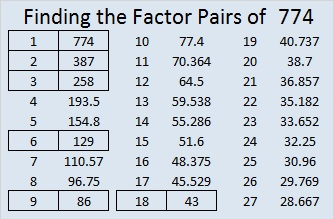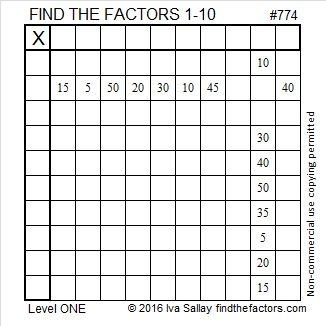# 774 and Level 1

• 774 is a composite number.
• Prime factorization: 774 = 2 x 3 x 3 x 43, which can be written 774 = 2 x (3^2) x 43
• The exponents in the prime factorization are 1, 2, and 1. Adding one to each and multiplying we get (1 + 1)(2 + 1)(1 + 1) = 2 x 3 x 2 = 12. Therefore 774 has exactly 12 factors.
• Factors of 774: 1, 2, 3, 6, 9, 18, 43, 86, 129, 258, 387, 774
• Factor pairs: 774 = 1 x 774, 2 x 387, 3 x 258, 6 x 129, 9 x 86, or 18 x 43
• Taking the factor pair with the largest square number factor, we get √774 = (√9)(√86) = 3√86 ≈ 27.820855.Here is a factoring puzzle that is just right for beginners:Print the puzzles or type the solution on this excel file: 10-factors-2016

———————-

Here’s a little more about the number 774:

774 is the sum of consecutive prime numbers two different ways:

• 11 + 13 + 17 + 19 + 23 + 29 + 31 + 37 + 41 + 43 + 47 + 53 + 59 + 61 + 67 + 71 + 73 + 79 = 774; that’s 18 consecutive primes.
• 29 + 31 + 37 + 41 + 43 + 47 + 53 + 59 + 61 + 67 + 71 + 73 + 79 + 83 = 774; that’s 14 consecutive primes.

774 is the sum of three triangular numbers eight different ways:

• 666 + 105 + 3 = 774
• 630 + 78 + 66 = 774
• 561 + 210 + 3 = 774
• 528 + 231 + 15 = 774
• 528 + 210 + 36 = 774
• 465 + 231 + 78 = 774
• 378 + 351 + 45 = 774
• 378 + 276 + 120 = 774

774 is also the sum of three squares nine different ways:

• 27² + 6² + 3² = 774
• 26² + 7² + 7² = 774
• 25² + 10² + 7² = 774
• 23² + 14² + 7² = 774
• 22² + 17² + 1² = 774
• 22² + 13² + 11² = 774
• 21² + 18² + 3² = 774
• 18² + 15² + 15² = 774
• 17² + 17² + 14² = 774

———————-This site uses Akismet to reduce spam. Learn how your comment data is processed.